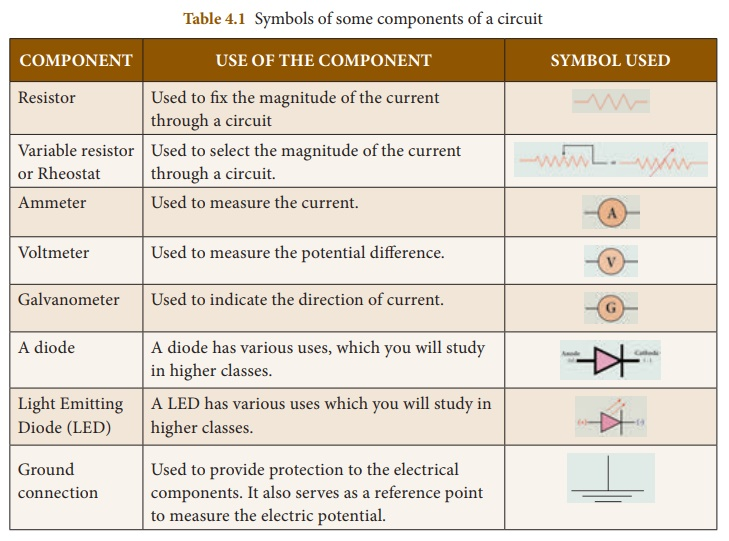Home | | Science 10th Std | Electric Circuit

# Electric Circuit

By convention, the direction of current is taken as the direction of flow of positive charge (or) opposite to the direction of flow of electrons. Thus, electric current passes in the circuit from the positive terminal to the negative terminal.

ELECTRIC CIRCUIT

An electric circuit is a closed conducting loop (or) path, which has a network of electrical components through which electrons are able to flow. This path is made using electrical wires so as to connect an electric appliance to a source of electric charges (battery). A schematic diagram of an electric circuit comprising of a battery, an electric bulb, and a switch is given in Figure 4.2.Figure 4.2 A simple electric circuitIn this circuit, if the switch is ŌĆśonŌĆÖ, the bulb glows. If it is switched off, the bulb does not glow. Therefore, the circuit must be closed in order that the current passes through it. The potential difference required for the flow of charges is provided by the battery. The electrons flow from the negative terminal to the positive terminal of the battery.

By convention, the direction of current is taken as the direction of flow of positive charge (or) opposite to the direction of flow of electrons. Thus, electric current passes in the circuit from the positive terminal to the negative terminal.

## Electrical components

The electric circuit given in Figure 4.2 consists of different components, such as a battery, a switch and a bulb. All these components can be represented by using certain symbols. It is easier to represent the components of a circuit using their respective symbols.

The symbols that are used to represent some commonly used components are given in Table 4.1. The uses of these components are also summarized in the table.

Study Material, Lecturing Notes, Assignment, Reference, Wiki description explanation, brief detail
10th Science : Chapter 4 : Electricity : Electric Circuit |